Sie sind hier: ICP » R. Hilfer » Publikationen

# 2 Relaxation functions and dielectric susceptibilities

[123.3.1] Let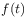denote the normalized, i.e.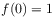, electrical dipolar polarisation or a similar relaxation function. [123.3.2] Then the complex frequency dependent dielectric susceptibility is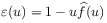, where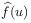denotes the Laplace transform of,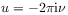,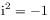, and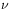is the frequency [16, p. 402, eq. (18)]. [123.3.3] Time honoured functional expressions forand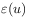are the exponential (Debye) relaxation [6, ch.III,§10]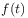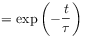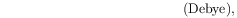(1a)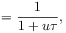(1b)

or stretched exponential Kohlrausch relaxation [26, 27], revived in  (KWW),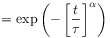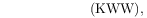(2a)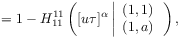(2b)

where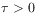is the relaxation time and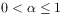is the stretching exponent. [123.3.4] Remarkably, while formula (2a) for the relaxation function has been used since 1854, formula (2b) for the dielectric susceptibility was discovered only in 2001 and published in . [123.3.5] It is given in terms of inverse Mellin-transforms of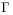-functions, also known as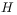-functions . [123.3.6] A brief definition is can be found in the Appendix below.

[123.4.1] A popular alternative to stretching time is to stretch frequency. [123.4.2] In this case a stretching exponent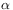is introduced into eq. (1b) rather than into eq. (1a). [123.4.3] This leads to the Cole-Cole (CC) relaxation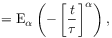(3a)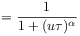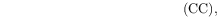(3b)

where(4)

is the Mittag-Leffler function . [123.4.4] It is by now well known, that the relaxation functionfor Cole-Cole relaxation is intimately related to fractional calculus . [123.4.5] Unfortunately, the Cole-Cole form (3b) exhibits a symmetric-peak, while asymmetric-peaks are observed experimentally for many materials . [123.4.6] Therefore, a second way to introduce the stretching exponentinto the Debye function (1b), known as the Cole-Davidson (CD) form, was introduced in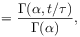(5a)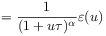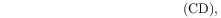(5b)

where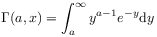(6)

denotes the complementary incomplete Gamma function, [123.4.7] Finally, the CC-form and CD-form are combined into the popular Havriliak-Negami (HN) form given as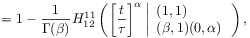(7a)(7b)

[123.4.8] Formula(7a) for the Havriliak-Negami relaxation function was first given in .

[123.5.1] The functional forms (1), (3), (5), and (7) are used universally almost without exception to fit broadband dielectric data. [123.5.2] A quantitative comparison of the different forms is shown for propylene carbonate at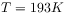in Figure 2. [123.5.3] It is found, that all of the functional forms (1), (3), (5), and (7) deviate from the experimetal data at high ferquency or give an unsatisfactory fit. [123.5.4] Therefore, a combination of two or more of these functional forms is routinely used to fit the excess wing in glass forming materials (see e.g. Figure 3.5 in [28, p.66]).